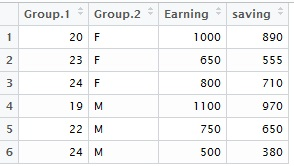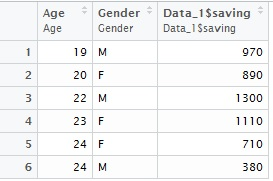## Pages

###R Tutorial 9.0

The blog covers the aggregation of data in R. In SAS we generally use Proc SQL or Proc Summary to do the same.

There are multiple packages  available in R for doing so,we shall explore most used one here.

First let's create a data which we would try to summarize.

rm(list = ls())   # Let's clear work space

Name = c("A","B","C","D","E","F","G","H")
Age = c(22,23,24,20,19,24,22,23)
Gender= c("M","F","M","F","M","F","M","F")
Earning= c(800,700,500,1000,1100,800,700,600)
Expense = c(100,110,120,110,130,90,100,80)

Data_1 = data.frame(Name, Age,Gender, Earning, Expense)
rm(Name,Age,Gender,Earning,Expense)
attach(Data_1)
Data_1\$saving = Earning - Expense
attach(Data_1)

### Let's now try to aggregate the data :

# First calculate total earning, expense and saving gender wise
Gender_wise_sum = aggregate(Data_1[4:6], by= list(Gender) ,FUN = sum, na.rm=TRUE)

# Now calculate mean earning and saving Age and gender wiseAge_Gender_wise_mean = aggregate(Data_1[c(4,6)], by= list(Age,Gender) ,FUN = mean, na.rm=TRUE)

The output columns are required to be renamed to make more sense (i.e. Group.1 and Group.2).

names(Age_Gender_wise_mean ) = "Age"
names(Age_Gender_wise_mean ) = "Gender"

na.rm = TRUE option basically tell R to not consider the missing values in the calculation.

by = list(x,y) option states x and y to be the class variables

FUN means function, we can specify sum, mean, sd, median, min, max, etc. based on requirement.

** sd stands for standard deviation.

If there all the numeric variables in the data, we need not tell the variables to be aggregated, it automatically aggregates all the numeric variables. But we recommend that you specify the columns to be aggregated.

### We can also use Hmisc packages for aggregation :

The function in this package is summarize.aggre = summarize(Data_1\$saving, llist(Age, Gender), sum)

The Data_1\$Saving column can then be renamed : names(aggre ) = "total_saving"

Enjoy reading our other articles and stay tuned with us.

Kindly do provide your feedback in the 'Comments' Section and share as much as possible.